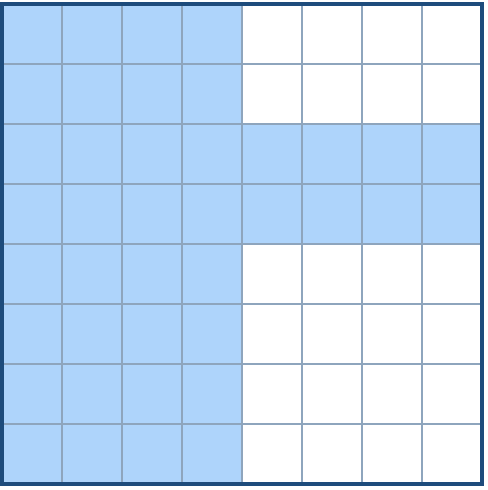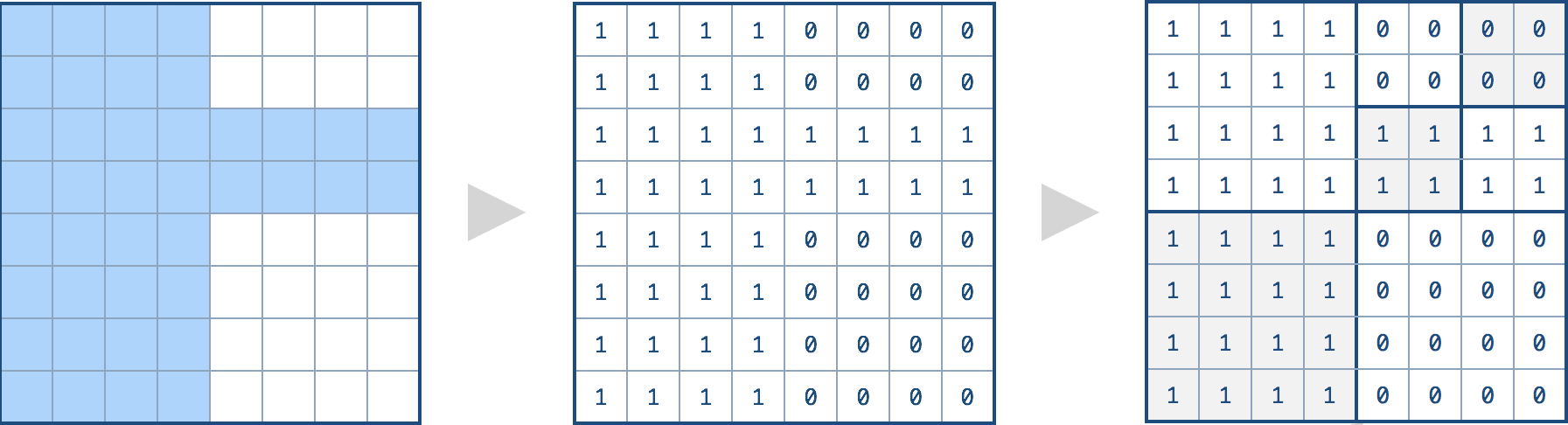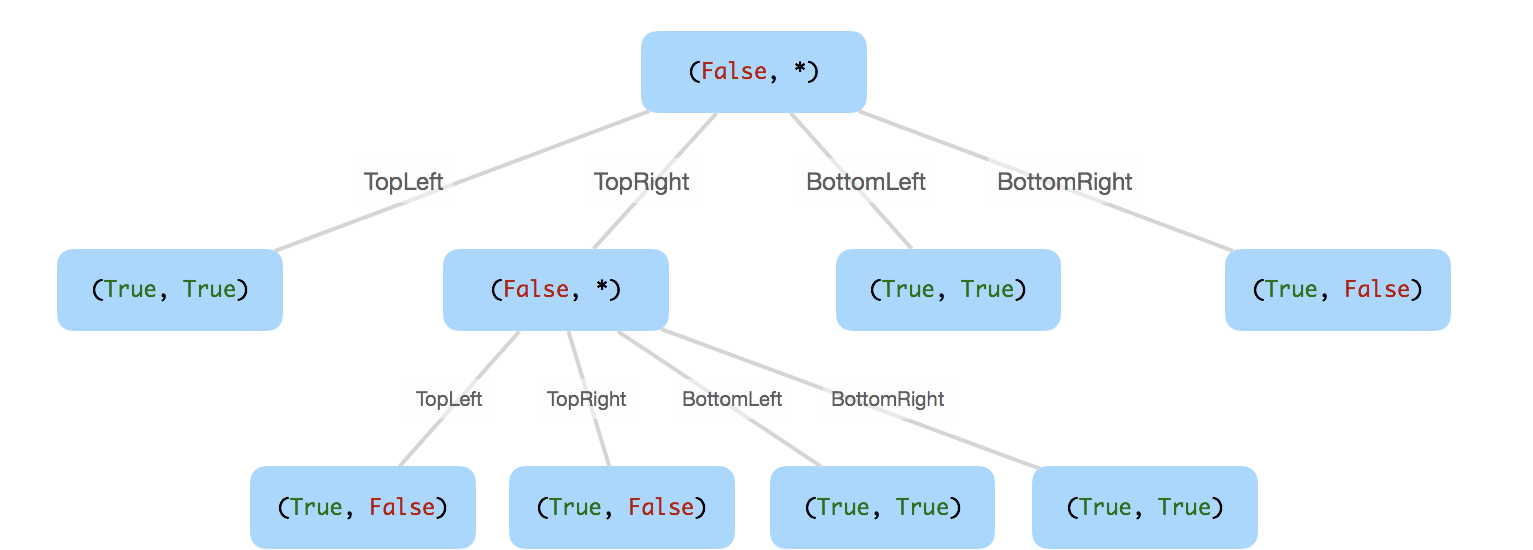##### Welcome to Subscribe On Youtube

Formatted question description: https://leetcode.ca/all/427.html

# 427. Construct Quad Tree (Easy)

We want to use quad trees to store an N x N boolean grid. Each cell in the grid can only be true or false. The root node represents the whole grid. For each node, it will be subdivided into four children nodes until the values in the region it represents are all the same.

Each node has another two boolean attributes : isLeaf and val. isLeaf is true if and only if the node is a leaf node. The val attribute for a leaf node contains the value of the region it represents.

Given the 8 x 8 grid below, we want to construct the corresponding quad tree:It can be divided according to the definition above:The corresponding quad tree should be as following, where each node is represented as a (isLeaf, val) pair.

For the non-leaf nodes, val can be arbitrary, so it is represented as *.Note:

1. N is less than 1000 and guaranteened to be a power of 2.
2. If you want to know more about the quad tree, you can refer to its wiki.

Companies:
Uber

## Solution 1.

// OJ: https://leetcode.com/problems/construct-quad-tree/
// Time: O(logN * N^2)
// Space: O(logN)
class Solution {
private:
Node *dfs(vector<vector<int>> &grid, int x, int y, int N) {
bool same = true;
for (int i = 0; i < N && same; ++i) {
for (int j = 0; j < N && same; ++j) {
same = grid[x][y] == grid[x + i][y + j];
}
}
if (same) return new Node(grid[x][y], true, NULL, NULL, NULL, NULL);
return new Node(true, false,
dfs(grid, x, y, N / 2),
dfs(grid, x, y + N / 2, N / 2),
dfs(grid, x + N / 2, y, N / 2),
dfs(grid, x + N / 2, y + N / 2, N / 2));
}
public:
Node* construct(vector<vector<int>>& grid) {
return dfs(grid, 0, 0, grid.size());
}
};


Java

• /*
// Definition for a QuadTree node.
class Node {
public boolean val;
public boolean isLeaf;
public Node topLeft;
public Node topRight;
public Node bottomLeft;
public Node bottomRight;

public Node() {}

public Node(boolean _val,boolean _isLeaf,Node _topLeft,Node _topRight,Node _bottomLeft,Node _bottomRight) {
val = _val;
isLeaf = _isLeaf;
topLeft = _topLeft;
topRight = _topRight;
bottomLeft = _bottomLeft;
bottomRight = _bottomRight;
}
};
*/
class Solution {
public Node construct(int[][] grid) {
if (grid == null || grid.length == 0 || grid.length == 0)
return null;
int length = grid.length;
if (length == 1) {
int gridVal = grid;
boolean val = gridVal == 1 ? true : false;
Node root = new Node(val, true, null, null, null, null);
return root;
} else {
int halfLength = length / 2;
int[][] topLeftGrid = new int[halfLength][halfLength];
int[][] topRightGrid = new int[halfLength][halfLength];
int[][] bottomLeftGrid = new int[halfLength][halfLength];
int[][] bottomRightGrid = new int[halfLength][halfLength];
for (int i = 0; i < halfLength; i++) {
for (int j = 0; j < halfLength; j++) {
topLeftGrid[i][j] = grid[i][j];
topRightGrid[i][j] = grid[i][j + halfLength];
bottomLeftGrid[i][j] = grid[i + halfLength][j];
bottomRightGrid[i][j] = grid[i + halfLength][j + halfLength];
}
}
Node topLeft = construct(topLeftGrid);
Node topRight = construct(topRightGrid);
Node bottomLeft = construct(bottomLeftGrid);
Node bottomRight = construct(bottomRightGrid);
if (topLeft.isLeaf && topRight.isLeaf && bottomLeft.isLeaf && bottomRight.isLeaf && topLeft.val == topRight.val && topLeft.val == bottomLeft.val && topLeft.val == bottomRight.val) {
Node root = new Node(topLeft.val, true, null, null, null, null);
return root;
} else {
boolean val = topLeft.val || topRight.val || bottomLeft.val || bottomRight.val;
Node root = new Node(val, false, topLeft, topRight, bottomLeft, bottomRight);
return root;
}
}
}
}

• // OJ: https://leetcode.com/problems/construct-quad-tree/
// Time: O(log_4^N * N^2)
// Space: O(log_4^N)
class Solution {
private:
Node *dfs(vector<vector<int>> &grid, int x, int y, int N) {
bool same = true;
for (int i = 0; i < N && same; ++i) {
for (int j = 0; j < N && same; ++j) {
same = grid[x][y] == grid[x + i][y + j];
}
}
if (same) return new Node(grid[x][y], true);
return new Node(true, false,
dfs(grid, x, y, N / 2),
dfs(grid, x, y + N / 2, N / 2),
dfs(grid, x + N / 2, y, N / 2),
dfs(grid, x + N / 2, y + N / 2, N / 2));
}
public:
Node* construct(vector<vector<int>>& grid) {
return dfs(grid, 0, 0, grid.size());
}
};

• """
# Definition for a QuadTree node.
class Node:
def __init__(self, val, isLeaf, topLeft, topRight, bottomLeft, bottomRight):
self.val = val
self.isLeaf = isLeaf
self.topLeft = topLeft
self.topRight = topRight
self.bottomLeft = bottomLeft
self.bottomRight = bottomRight
"""

class Solution:
def construct(self, grid: List[List[int]]) -> 'Node':
def dfs(a, b, c, d):
zero = one = 0
for i in range(a, c + 1):
for j in range(b, d + 1):
if grid[i][j] == 0:
zero = 1
else:
one = 1
isLeaf = zero + one == 1
val = isLeaf and one
if isLeaf:
return Node(grid[a][b], True)
topLeft = dfs(a, b, (a + c) // 2, (b + d) // 2)
topRight = dfs(a, (b + d) // 2 + 1, (a + c) // 2, d)
bottomLeft = dfs((a + c) // 2 + 1, b, c, (b + d) // 2)
bottomRight = dfs((a + c) // 2 + 1, (b + d) // 2 + 1, c, d)
return Node(val, isLeaf, topLeft, topRight, bottomLeft, bottomRight)

return dfs(0, 0, len(grid) - 1, len(grid) - 1)

############

"""
# Definition for a QuadTree node.
class Node:
def __init__(self, val, isLeaf, topLeft, topRight, bottomLeft, bottomRight):
self.val = val
self.isLeaf = isLeaf
self.topLeft = topLeft
self.topRight = topRight
self.bottomLeft = bottomLeft
self.bottomRight = bottomRight
"""
class Solution:
def construct(self, grid):
"""
:type grid: List[List[int]]
:rtype: Node
"""
_len = len(grid)
if isLeaf == None:
mid = _len // 2
topLeftGrid = [[grid[i][j] for j in range(mid)] for i in range(mid)]
topRightGrid = [[grid[i][j] for j in range(mid, _len)] for i in range(mid)]
bottomLeftGrid = [[grid[i][j] for j in range(mid)] for i in range(mid, _len)]
bottomRightGrid = [[grid[i][j] for j in range(mid, _len)] for i in range(mid, _len)]
node = Node(True, False, self.construct(topLeftGrid), self.construct(topRightGrid),
self.construct(bottomLeftGrid), self.construct(bottomRightGrid))
elif isLeaf == False:
node = Node(False, True, None, None, None, None)
else:
node = Node(True, True, None, None, None, None)
return node

_len = len(grid)
_sum = 0
for i in range(_len):
_sum += sum(grid[i])
if _sum == _len ** 2:
return True
elif _sum == 0:
return False
else:
return None